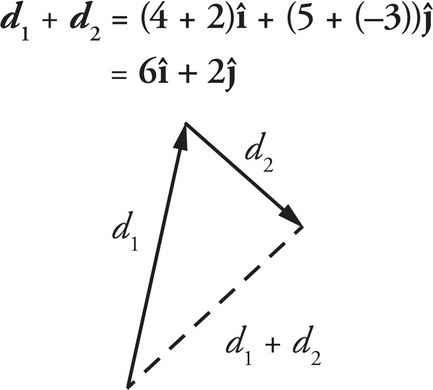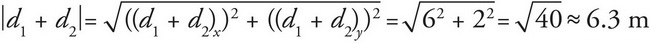# AP Physics 1 Question 187: Answer and Explanation

### Test Information

Question: 187

7. An object travels along the vector d1 = 4m î + 5m ĵ and then along the vector d2 = 2m î - 3m ĵ. How far is the object from where it started?

• A. 6.3 m
• B. 8 m
• C. 10 m
• D. 14 m

Explanation:

A

If the object travels along the vectors d1 and then d2, then the total distance travelled with be the sum of the two vectors d1 + d2:The total distance of the object from the starting position is therefore the magnitude d1 + d2:That's (A).